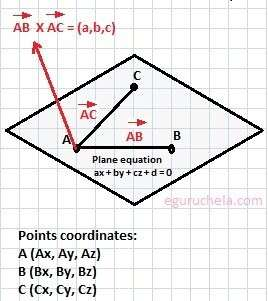### Cartesian Equation of the Plane Calculation

Calculate the equation of a three-dimensional plane in space for entered values.
Suppose the normal vector is defined as n = < A, B, C> where n is a vector, and A, B, and C are scalars.Please enter the coordinates of :
 Point A : , , Point B : , , Point C: , , Equation of the plane: x y z =0

There are different methods to define a plan like; a point and normal vector, three non-collinear points, a line and a point not on the line, two parallel and non-coincident line and two intersecting lines. The Cartesian equation of a plane is ax + by + cy + d = 0 where a,b and c are the vector normal to the plane. A Cartesian coordinate system for three-dimensional space plane has three axis(x, y, and z).
We can determine the equation of the plane that contains the 3 point in the xyz-coordinate in following form:
ax + by + cz + d = 0
where a, b, c, and d are real numbers and derivation is as follows:
a = (By-Ay)(Cz-Az)-(Cy-Ay)(Bz-Az)
b = (Bz-Az)(Cx-Ax)-(Cz-Az)(Bx-Ax)
c = (Bx-Ax)(Cy-Ay)-(Cx-Ax)(By-Ay)
d = -(aAx+bAy+cAz).
Let's take the example where all the 3 points are as follows in three-dimensional space: